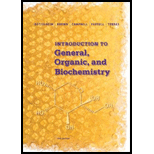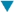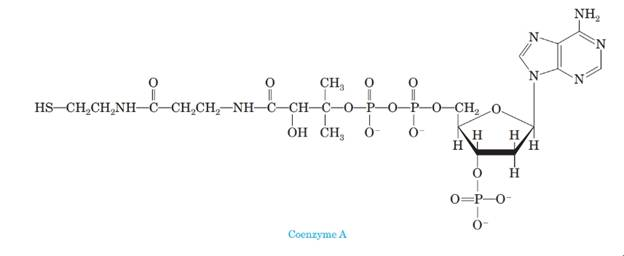# 4 Below is the structural formula of coenzyme A, an important biomolecule. (a) Is coenzyme A chiral? (b) Name each functional group in coenzyme A. (c) Would you expect coenzyme A to be soluble in water? Explain. (d) Draw structural formulas for the products of complete hydrolysis of coenzyme A in aqueous HCI. Show each product as it would be ionized in this solution. (e) Draw structural formulas for the products of complete hydrolysis of coenzyme A in aqueous NaOH. Show each product as it would be ionized in this solution.### Introduction to General, Organic a...

11th Edition
Frederick A. Bettelheim + 4 others
Publisher: Cengage Learning
ISBN: 9781285869759

#### Solutions

Chapter
Section### Introduction to General, Organic a...

11th Edition
Frederick A. Bettelheim + 4 others
Publisher: Cengage Learning
ISBN: 9781285869759
Chapter 20, Problem 20.84P
Textbook Problem
1 views

## 4 Below is the structural formula of coenzyme A, an important biomolecule.(a) Is coenzyme A chiral?(b) Name each functional group in coenzyme A.(c) Would you expect coenzyme A to be soluble in water? Explain.(d) Draw structural formulas for the products of complete hydrolysis of coenzyme A in aqueous HCI. Show each product as it would be ionized in this solution.(e) Draw structural formulas for the products of complete hydrolysis of coenzyme A in aqueousNaOH. Show each product as it would be ionized in this solution.Interpretation Introduction

(a)

Interpretation:

Whether coenzyme A is chiral is to be predicted.

Concept Introduction:

Coenzymes are derived from Vitamins. They are the substance required in small amounts for the normal functioning of the body that the body cannot synthesize in adequate amounts. They are non-proteins molecules which help enzymes for catalyzing the reaction.

A carbon atom that has four nonequivalent atoms or groups attached to it is known as chiral carbon atom. Chiral carbon centers are also called as asymmetric or stereogenic centers.

### Explanation of Solution

The structure of coenzyme A is shown below.

Figure 1.

A carbon atom that has four nonequivalent atoms or groups attached to it is known as chiral carbon atom. In coenzyme A, chiral carbon atom is present...

Interpretation Introduction

(b)

Interpretation:

The names of each functional group in coenzyme A are to be stated.

Concept Introduction:

Coenzymes are derived from Vitamins. They are the substance required in small amounts for the normal functioning of the body that the body cannot synthesize in adequate amounts. They are non-proteins molecules which help enzymes for catalyzing the reaction.

An atom or a group of atoms that show characteristic physical and chemical properties are collectively known as functional groups. The functional group is the most reactive part present in the molecule. The main functional groups are: OH (alcohol group), COOH (carboxylic acid group), CHO (aldehyde group) and C=O (ketone group).

Interpretation Introduction

(c)

Interpretation:

An explanation corresponding to the solubility of coenzyme A in water is to be stated.

Concept Introduction:

Coenzymes are derived from Vitamins. They are the substance required in small amounts for the normal functioning of the body that the body cannot synthesize in adequate amounts. They are non-proteins molecules which help enzymes for catalyzing the reaction.

Interpretation Introduction

(d)

Interpretation:

The structural formulas for the products of the complete hydrolysis of coenzyme A in aqueous HCl solution showing the ionization of each product are to be drawn.

Concept Introduction:

Coenzymes are derived from Vitamins. They are the substance required in small amounts for the normal functioning of the body that the body cannot synthesize in adequate amounts. They are non-proteins molecules which help enzymes for catalyzing the reaction.

Interpretation Introduction

(e)

Interpretation:

The structural formulas for the products of the complete hydrolysis of coenzyme A in aqueous NaOH solution showing the ionization of each product are to be drawn.

Concept Introduction:

Coenzymes are derived from Vitamins. They are the substance required in small amounts for the normal functioning of the body that the body cannot synthesize in adequate amounts. They are non-proteins molecules which help enzymes for catalyzing the reaction.

### Still sussing out bartleby?

Check out a sample textbook solution.

See a sample solution

#### The Solution to Your Study Problems

Bartleby provides explanations to thousands of textbook problems written by our experts, many with advanced degrees!

Get Started

Find more solutions based on key concepts
Name the following molecular compounds a ClF4 b CS2 c PF3 d SF6

General Chemistry - Standalone book (MindTap Course List)

Magnesium a. assists in the operation of enzymes. b. is needed for the release and use of energy. c. is critica...

Nutrition: Concepts and Controversies - Standalone book (MindTap Course List)

What are the chemical differences between DNA and RNA?

Biology: The Dynamic Science (MindTap Course List)

At one location on the Earth, the rms value of the magnetic field caused by solar radiation is 1.80 T. From thi...

Physics for Scientists and Engineers, Technology Update (No access codes included)